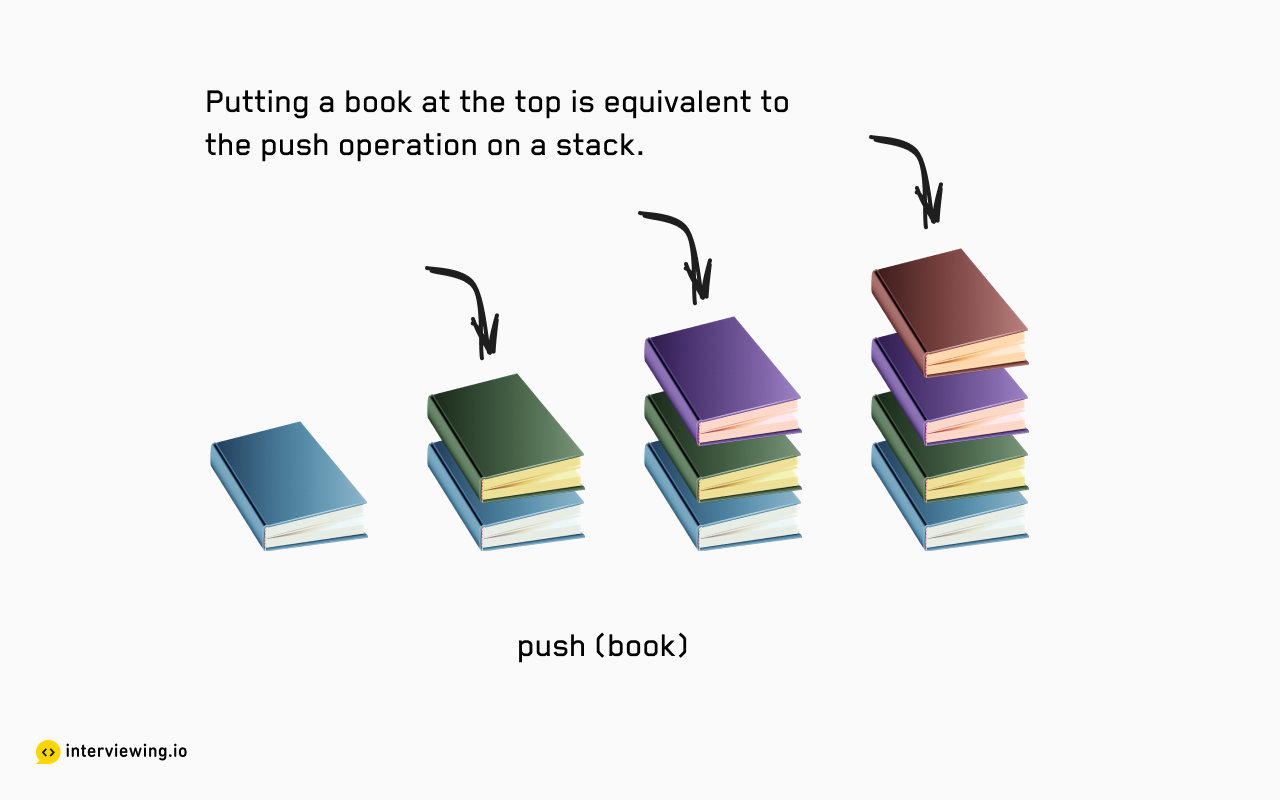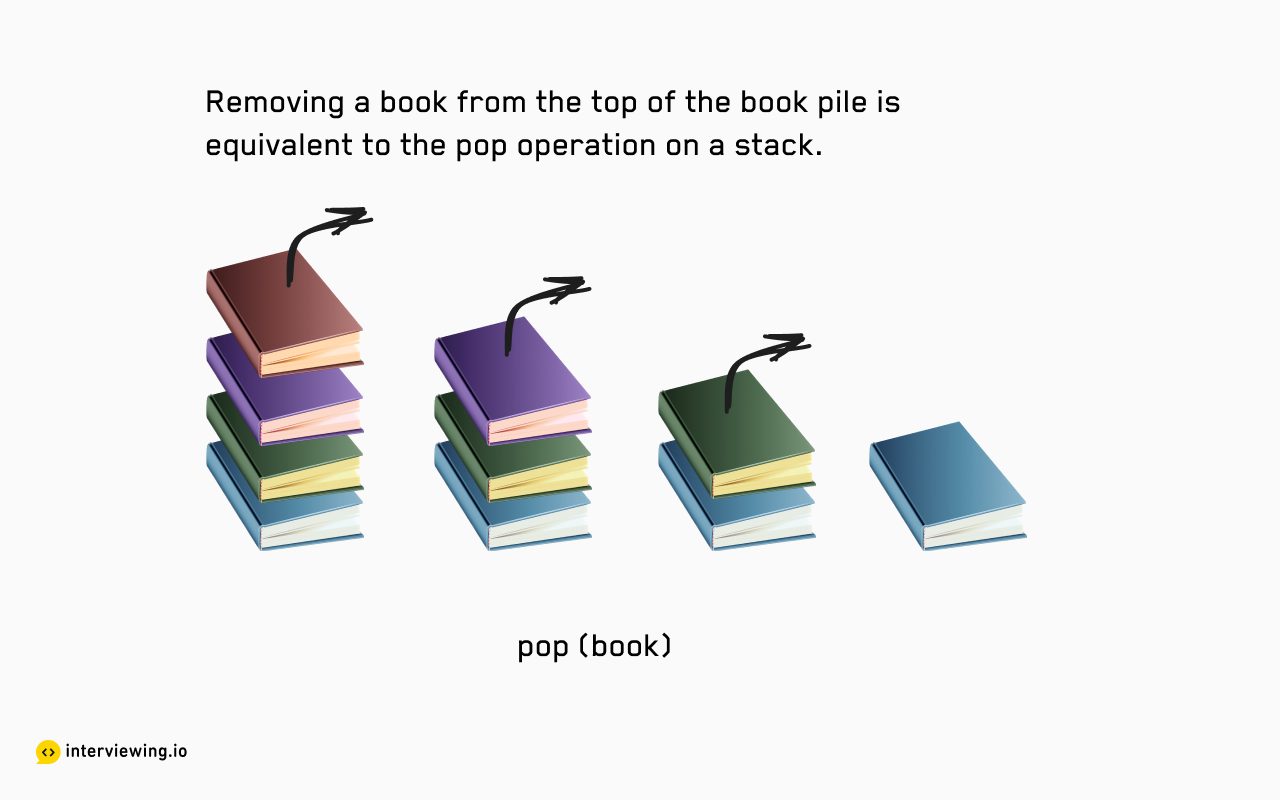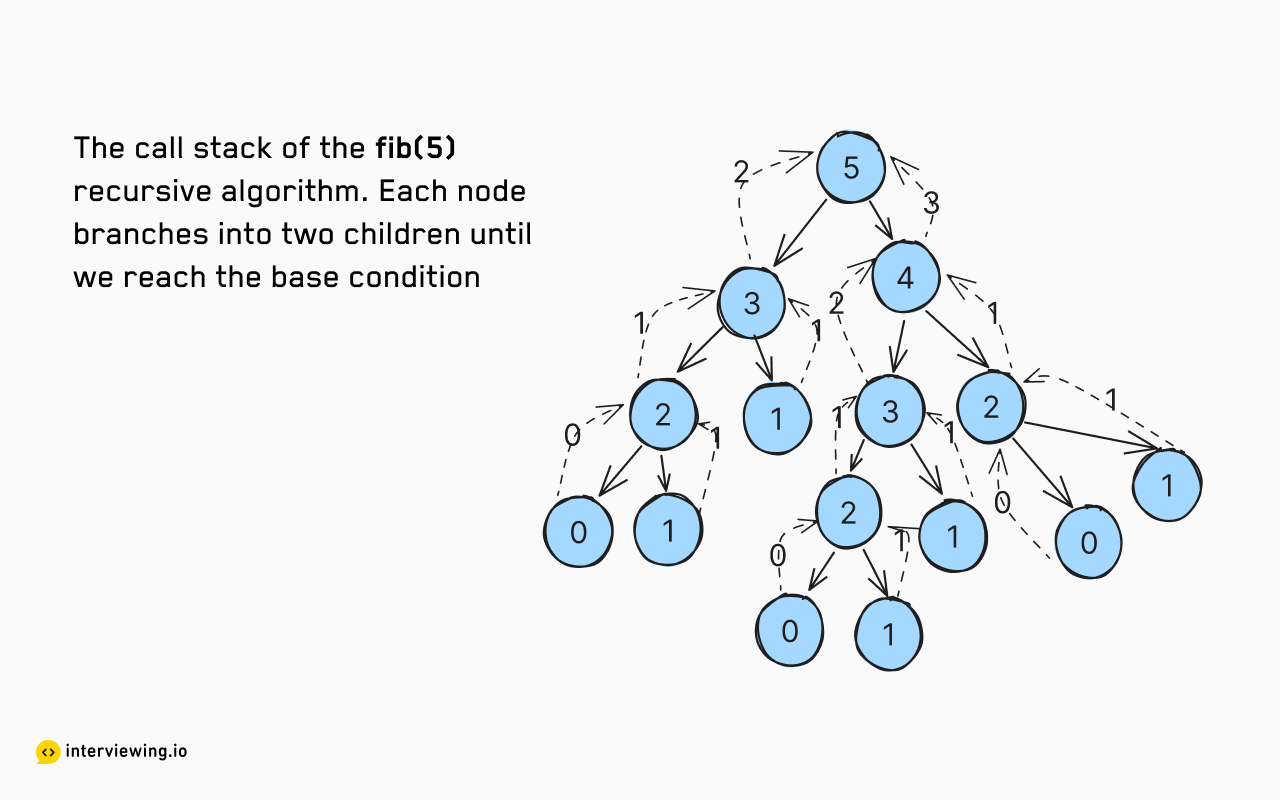# Stacks Interview Questions & Tips

## What is a Stack?

A stack is a linear data structure that permits access to its underlying data through two primary operations: 'push' (to add) and 'pop' (to remove).

Let's visualize how a stack operates with an analogy. Consider the desk of my history professor, always brimming with a pile of books. The most recently acquired book occupies the top spot in this stack. If he wants to revisit an older book, he must take off all the books above it, one at a time. Once he retrieves his desired book, he has to replace the rest, with the latest one reclaiming its position on top. This act of taking off a book from the top mirrors the 'pop' operation while placing a new book onto the stack is akin to 'push'. The stack data structure uniquely allows for the addition or removal of data from only one end, much like this pile of books.### Stack Compared to a Queue

A queue provides a similar linear data structure that differs from a stack only in how it is accessed. A stack adds and removes items from the same end, while in the case of a queue, an item is added to one end and removed from the other. This is why a queue is commonly called a FIFO structure (First In First Out).

### Stack Operations

A stack supports the following operations:

• `push`: adds an item to the top of the stack
• `pop`: removes the top item from the stack
• `peek`: returns the top item from the stack without removing it
• `isEmpty`: returns true if the stack is empty, false otherwise
• `size`: returns the number of items in the stack

The stack is a key player in computer science, powering many core algorithms. It's behind everyday tasks like clicking a 'back' button in a browser or using 'undo' and 'redo' in a document. Operating systems use stacks to keep track of running processes and to store local variables. Compilers use them to evaluate expressions and parse syntax. And, as we'll see in this article, interviewers love to ask questions about stacks.

## When to Use Stacks in Interviews

Stacks are a great way to test a candidate's understanding of fundamental data structures, so the topic is frequently covered in interviews, although chances are the interviewer won't directly spell out a 'stack' for you to implement. Instead, they'll present you with a problem that can be solved using a stack. So, it's important to be able to recognize such problems and to know how to apply a stack to solve them. Some things which should trigger the use of a stack are: changing the order of a given sequence, dealing with nested structures like parentheses, or a recursive algorithm. Let's look at some examples below.

#### When you are asked to reverse the order of a given sequence

You are given a string, and you need to reverse it. For example, given the string "hello", you should return "olleh".

Whenever you encounter a problem that involves reversing the order of something, such as a string or an array, the stack data structure should be your go-to solution. In such scenarios, the approach would be to push each character of the string or each element of the array onto a stack, and then pop them off one by one. This will result in the elements being reversed, demonstrating the power and usefulness of stacks in such situations.

Note that most of the time, when you’re asked to reverse a string, you’ll be doing it in place. While the in-place solution offers superior space complexity, sometimes you might have to NOT do it in place, and that’s when a stack-based approach still serves as an excellent starting point.

``````def reverse_string(string):
stack = []
for char in string:
# iterate over each character in the string and push
# it onto the stack
stack.append(char)
reversed_string = []
while len(stack) > 0:
# pop each character off the stack and append to the
# reversed_string list. The list will later be joined
# to form a string
reversed_string.append(stack.pop())
return "".join(reversed_string)

print(reverse_string("hello"))  # prints: olleh``````

Although this might look like a good answer, the interviewer may want to probe you further to see if you can come up with a more optimal solution. The above solution has a space complexity of `O(n)` because we use an extra stack to store the characters. The in-place solution does it all without using extra space, bringing the space complexity down to `O(1)`.

### When You're Asked to Validate a Sequence of Brackets

You are given a string containing only parentheses, brackets, and curly braces. You need to determine if the string is valid. A string is considered valid if all the brackets are closed in the correct order. For example, "([])" is valid, but "([)]" is not.

This is another classic stack question. The solution is to push each opening bracket onto a stack and then pop them off when a closing bracket is encountered. If the closing bracket doesn't match the opening bracket at the top of the stack, the string is invalid.

``````def is_balanced(s):
stack = []
brackets = {"(": ")", "{": "}", "[": "]"}

for char in s:
# If the character is an opening bracket, push it onto the stack
if char in brackets:
stack.append(char)
else:
# If the stack is empty, it means there is no opening bracket
# for the current closing bracket, so return False
if not stack:
return False
# If the top of the stack doesn't match the current character, return False
elif brackets[stack.pop()] != char:
return False

return not stack

print(is_balanced("({[]})"))  # prints: True
print(is_balanced("({[}))"))  # prints: False``````

Takeaway: When you are asked to validate a nested structure (like parentheses in this case), think of using a stack.

### When You're Asked to Implement a Recursive Algorithm Iteratively

In a recursive algorithm, a function calls itself until it reaches a base case. The recursive calls are stored in a stack, although this stack is implicitly maintained by the system and is invisible to the programmer. So to convert a recursive algorithm to an iterative one, you need to simulate the recursive call stack using an explicit stack.

Say, you are tasked to implement Depth First Search on a binary tree. The recursive solution is pretty straightforward. It involves five lines of code. The interviewer might ask you to implement it iteratively. As suggested earlier, you can use a stack here.

``````# recursive
def dfs(root):
if not root:
return
dfs(root.left)
dfs(root.right)

# iterative
def dfs(root):
stack = [root]
while stack:
node = stack.pop()
if node.right:
stack.append(node.right)
if node.left:
stack.append(node.left)``````

### When You're Asked to Find the Next Greater (or Smaller) Element in a Sequence

This category of problems can be solved using a monotonic stack. A monotonic stack is a stack that is either strictly increasing or decreasing. In other words, the elements in the stack are sorted in either ascending or descending order. For the next greater element, we make a monotonically decreasing stack. For the next smaller element, we make a monotonically increasing stack.

``````def next_greater_element(nums):
result = [-1] * len(nums)
stack = []
# iterate over the array
for i in range(len(nums)):
# if the current element is greater than the
# element at the top of the stack, pop the stack
# the current element is the next greater element
# for all the elements in the stack which are less
while stack and nums[stack[-1]] < nums[i]:
result[stack.pop()] = nums[i]
stack.append(i)
return result

print(next_greater_element([2, 1, 2, 4, 3]))  # prints: [4, 2, 4, -1, -1]``````

### When You're Asked to implement a Queue Using Stacks

Typically a queue is implemented using a linked list. But it can also be implemented using two stacks.

One stack is used to enqueue elements, and the other is used to dequeue elements. When the dequeue stack is empty, we pop all the elements from the enqueue stack and push them onto the dequeue stack. This reverses the order of the elements, giving us the FIFO (First In First Out) property of a queue.

``````class Queue:
def __init__(self):
self.enqueue_stack = []
self.dequeue_stack = []

def enqueue(self, x):
# append the new element to the enqueue stack
self.enqueue_stack.append(x)

def dequeue(self):
# if dequeue stack is empty pop all the elements
# from enqueue stack and push them onto the dequeue stack
if not self.dequeue_stack:
while self.enqueue_stack:
self.dequeue_stack.append(self.enqueue_stack.pop())
# pop the element from the dequeue stack and return it
return self.dequeue_stack.pop()``````

This solution might follow a discussion on the design choices made and time complexity of this approach.

Enqueue operations, implemented as push operations on the enqueue stack, have a time complexity of `O(1)`. Dequeue operations, however, are more intricate. In the worst case, when the dequeue stack is empty, all items are transferred from the enqueue stack, resulting in a time complexity of `O(n)`. In the best case, where the dequeue stack is not empty, the item is popped in `O(1)`. Over many operations, the time complexity averages out to an amortized `O(1)`. This is because each element undergoes at most three stack interactions. First, each element is subjected to an enqueue operation. Then, it is moved from the enqueue stack to the dequeue stack. Finally, a dequeue operation is performed on the element.

### Concurrency problems

In concurrent programming, data structures like stacks often play a crucial role, particularly in the classic producer-consumer problem. This problem involves two types of processes: the producer, which generates data and adds it to the stack, and the consumer, which removes and processes data from the stack.

To ensure smooth operation, the stack must be thread-safe, meaning multiple threads can execute push and pop operations without risking inconsistencies, known as race conditions. This thread safety is usually achieved by employing synchronization mechanisms like locks, which allow only one thread to modify the stack at any given time.

## Common Mistakes in Interviews Featuring Stacks

### Not Knowing the Time Complexity of Stack Operations

Stack operations are `O(1)` time because the underlying data structure is a linked list. Pushing and popping from the head of a linked list is `O(1)` time. Now, depending on which programming language you use, there might be differences in how the stack is implemented under the hood, these differences can affect your time complexity calculations. For example, in Python, a stack is implemented using a list, which in turn, is implemented as a dynamic array. When we need to add a lot of data to the stack, the underlying dynamic array may need to resize itself, which can be an expensive operation. To mitigate this cost, we can use deque from the collections module. A deque is implemented using a doubly linked list in Python and therefore doesn't need to resize itself.

``````rom collections import deque

stack = deque()

for i in range(100000):
stack.append(i)

stack.pop()``````

JavaScript, Go, and Ruby employ dynamic arrays for stack implementation; JavaScript uses Array, Go utilizes a slice, and Ruby adopts Array. All these constructs potentially experience `O(n)` time complexity during resizing due to their dynamic array nature.

### Not Considering the Space Complexity of Using the Implicit Stack for Recursive Algorithms

Recursive algorithms use the call stack to store intermediate results. This is an implicit stack, and it's important to consider its space complexity. For example, the recursive solution to the Fibonacci sequence has a space complexity of O(n). This is because the call stack will have at most n frames. Each frame corresponds to a recursive call to the function.

``````def fib(n):
if n <= 1:
return n
# each recursive call to fib() adds a frame to the call stack
return fib(n - 1) + fib(n - 2)``````

It usually helps to draw a recursive tree to visualize the call stack. The depth of the tree is the space complexity of the algorithm.### Not Checking for Stack Underflow

Stack underflow occurs when you try to pop an element from an empty stack. This is a common mistake. Most languages provide a version of checking whether the stack is empty before popping an element. For example, in Python, you can use the `if stack` check to see if the stack is empty before popping an element.

## Demonstrating Mastery Over Stacks in Interviews

### Knowing the Internal Implementation of a Stack

Stacks are commonly implemented using a linked list or a dynamic array. If this comes up during the interview, you should be able to explain the tradeoffs between the two approaches. For example, a linked list implementation is more space efficient because it doesn't need to resize itself. However, it's less cache-friendly because the elements are not stored contiguously in memory. A dynamic array implementation is less space efficient because it needs to resize itself. However, its cache behavior is friendlier because the elements are stored contiguously in memory.

#### Knowing Language-Specific Nuances

In Java, you can use the `Stack` class to implement a stack. However, it's recommended to use the `Deque` interface instead because the `Stack` class extends the `Vector` class, which is a legacy class. In Python, you can use the `list` class to implement a stack. However, you can also use the `deque` class from the `collections` module. In a multi-threaded environment, `deque` is a better choice because it's thread-safe and memory efficient. In JavaScript, you can use the `Array` class to implement a stack.

### Discussing the Space and Time Complexity of Stack Operations

As discussed earlier, recursion uses the call stack to store intermediate results. This implicit call stack results in higher space requirements and might end up causing a stack overflow. When you offer a solution, you can discuss the time and space complexities of your solution using an implicit or an explicit stack. Having the tradeoffs in mind will help you develop a better solution.

### Suggest ing an Iterative solution to a Recursive Algorithm

Most recursive algorithms can be easily converted to an iterative solution using a stack. There are distinct advantages to using an iterative solution: space savings and no risk of stack overflow. So, it's important to know the distinction. Sometimes, the interviewer may ask you not to use iteration because the recursive solution is more elegant. In that case, you can mention the iterative solution and explain why it's better.